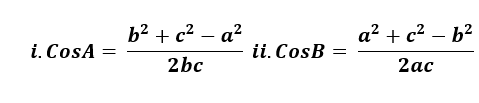# The Cosine Law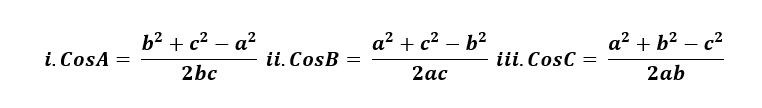Proof:
↪ We consider a triangle ABC then we can have three possible figures where angle C is acute in figure i, right angle in figure ii and obtuse in figure iii.From A draw AD perpendicular to BC ( Produce BC if necessary )

In ΔABC, AB2 = AD2 + BD2
⇒c2 = AD2 + BD → Ⅰ

In figure (i),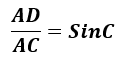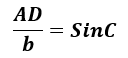⇒ AD = bSinC

Also,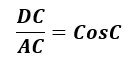⇒ DC = ACCosC = bCosC
⇒ BD = BC -  DC = a-bCosC

Now from equation Ⅰ,
c2 = AD2 + BD2 (becomes)
c2 = (bSinC)2 + (a-bCosC)2
c2 = b2Sin2C + a2 - 2abCosC + b2CosC2
c2 = b2 + a2 - 2abCosC
⇒ 2abCosC = b2 + a2 - c2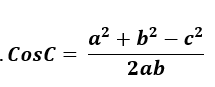Similarly in figure ii,

AB2 = BC2 + AC2
c2 = a2 + b2 = a2 + b2 - 2abCosC
2abCosC = a2 + b2 - c2And in figure iii,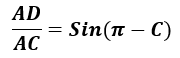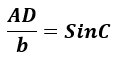⇒ AD = bSinC

Also,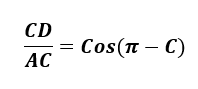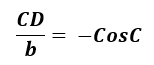⇒ CD = -bCosC
⇒ BD = BC + CD = a - bCosC

Now from equation Ⅰ,
c2 = AD2 + BD2
= (bSinC)2 + (a-bCosC)2
= b2Sin2C + a2 + b2Cos2C - 2abCosC
= b2 + a2 - 2abCosC
⇒ 2abCosC = b2 + a2 - c2Finally in all figures, it is seen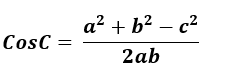Similarly we can show